# Arrays

## Arrays | Why we should learn Arrays

In this article, I am going to revising the concept of C and C++ language that is going to be used in this Data Structure and Algorithm course. Basically, this course is using the C language. But I am also going to show you how to write the code using C++. So, if you know C++, then It is an added advantage for you, even if you don’t know C++ it is sufficient to know the C language.

You should be already knowing the basics of C Programming and the concepts of C programming language that are frequently used in this course I am going to revise them, so that you are well acquainted or well familiar with those concepts and you can easily understand the rest of the topics.

##### So, in this section I’ll be going to discuss: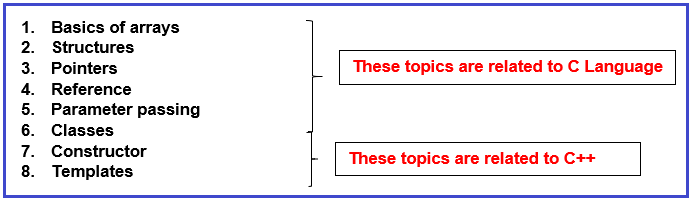The above-mentioned topics are going to be discussed in this section. If you are already well familiar with the C++ programming language & you are a good programmer, I suggest you go through this section so that you can understand the style of programming that I have adopted in this course.

So, let’s start with the Array. Now I’ll discuss a little bit about array & after that, we’ll cover all the topics one by one from our next article.

##### Arrays:

The array is defined as a collection of similar data elements. If you have some sets of integers, some sets of floats, you can group them under one name as an array.

##### Method of declaring an array

If you want an integer type array, let’s say int A;

Then, you will get 5 integers and the array name as “A” and you can all those integers using the name “A”. So, “A” is an array and you get 5 integers spaces and the indices will be 0,1,2,3,4. So, 5 location means indices will starts from 0 and end at 4, its total 5 spaces. For better understanding, please have a look at the below image.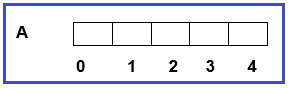Now we can store 5 integers. Every location is an integer. If we assume an integer is taking 2 bytes then these are total 10 bytes i.e. 2 bytes each. Now each location can be accessed like A=27; //Here, 27 will store in array “A” with indices 0. Now if I store A=10; //10 is stored in a place with indices 1. For better understanding, please have a look at the below image,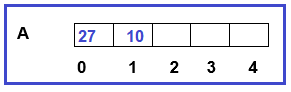So, we can have a group of elements in a single place.

##### How to declare and initialize an array?

Now I’ll show you how to declare and initialize an array. In the main function, suppose I want to declare an array “A” of size 5. Then you need to declare the array as shown below.

```int main()
{
int A;
}
```

Now, an array of size 5 will be created. When a program is running, then it is running inside the main memory. The main memory is divided into 3 sections i.e. Code section, Stack section & Heap section as shown in the below image.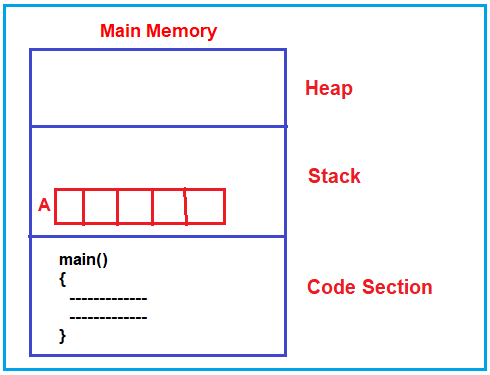As you can see in the above image, whatever code we write will be inside the code section. The point that you need to understand is, the variables that we declared, will be created inside the Stack section. So, here the Array is also going to be created inside the Stack as the array is also a variable.

Those variables that are created inside the stack section are directly accessed by the Main method from the code section. So, the array is directly accessible to the main function and can directly store the values in the array. Now, I hope you understand how the array is declared and where the array is created inside the main memory.

##### How to declare and initialize an array?

You can declare and initialize an array in the same line as shown in the below example.

```int main()
{
int A={1,3,5,7,9};
}
```

The above code shows the declaration of an array with size 5, along with this, the array is also initialized with the values 1,3,5,7,9. So, this is a declaration as well as the initialization of an array. The memory representation of the above is shown below.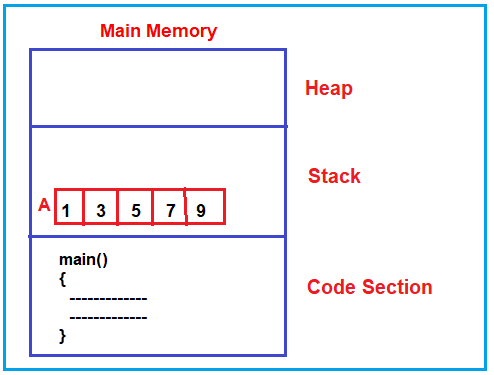##### How to access an array?

We can access all the elements of an array one by one using a “for” loop. To understand this better please have a look at the following code.

```int main()
{
int A={2, 4,6,8,10};
int i;
for(i=0;i<5;i++)
{
printf("%d",A[i]);
}
}
```

This is sufficient at this moment. Because we have a very big topic called arrays and we will be going to learn lots and lots of things about arrays.

Just as an introduction, the points that I have discussed here are what does it mean by an array and how to declare and initialize it and when it is declared inside a function then where it will appear inside the main memory.

In the next article, I am going to discuss Structures in detail. Here, in this article, I just give an overview of Arrays and I hope you enjoy this Arrays article.